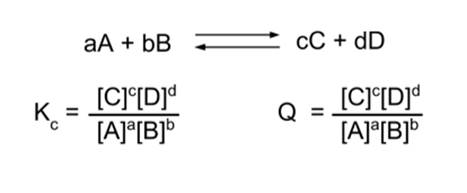# Enduring Understanding 6.A: Reversible Reactions

• Chemical reactions are reversible at a molecular level - they can proceed in either direction.
• When a reaction and its reverse are happening at the same rate, such that the concentration of reactants and products are not changing over time, this is called dynamic chemical equilibrium.
• Chemical equilibrium can be expressed mathematically:
•• When the reaction is in equilibrium, the concentrations of products and reactants can be used to calculate the equilibrium constant, Kc. The c denotes concentration; an equilibrium constant that uses pressure is Kp.
• Example : The reaction:
• SO2Cl2 (g) ⇆ SO2 (g) + Cl2 (g)
• At equilibrium in a rigid container, the concentration of SO2 and Cl2 is 0.085 mol/L and the concentration of SO2Cl2 is 0.915 mol/L. The Kc can be calculated:
• Kc = [SO2] [Cl2] / [SO2Cl2] = (0.085)(0.085)/(0.915) = 0.0079
• The reaction quotient is similar, but for situations in which the reaction mixture is NOT at equilibrium.
• Example : If 0.50 moles of SO2Cl2 and 0.20 moles of SO2 and Cl2 are combined in a 1L container, Q can be calculated:
• Qc = [SO2] [Cl2] / [SO2Cl2] = (0.20) (0.20) / (0.50) = 0.080
• Q and K can be compared, to determine which direction a chemical reaction will go in.
• If K > Q, the reaction will proceed in the forward direction.
• If K < Q, the reaction will proceed in the reverse direction.
• When K = Q, the reaction is at equilibrium. The rate of the forward and reverse reactions are equal.
• Note that only reactants that can change concentration or pressure contribute to the calculation of a K or a Q.
• Solid and liquid reagents are not included in equilibrium constant calculations.
• Example: The reaction quotient for the reaction:
• 2 ClO (s) ⇆ Cl2(g) + O2(g)
• Is given by:
• Q = [Cl2] [O2]
• The solid reagent ClO, cannot change in concentration, so it is not included in the equation.
• Similarly, the Kp for the reaction P4 (g) + 6F2 (g) ⇆ 4PF3 (l) is given by 1/[PP4] [PF2]6. The liquid product does not have a pressure, so cannot contribute to the pressure equilibrium constant.

 Related Links: Chemistry Chemistry Quizzes AP Chemistry Notes Gibbs Free Energy

To link to this Reversible Reactions page, copy the following code to your site: